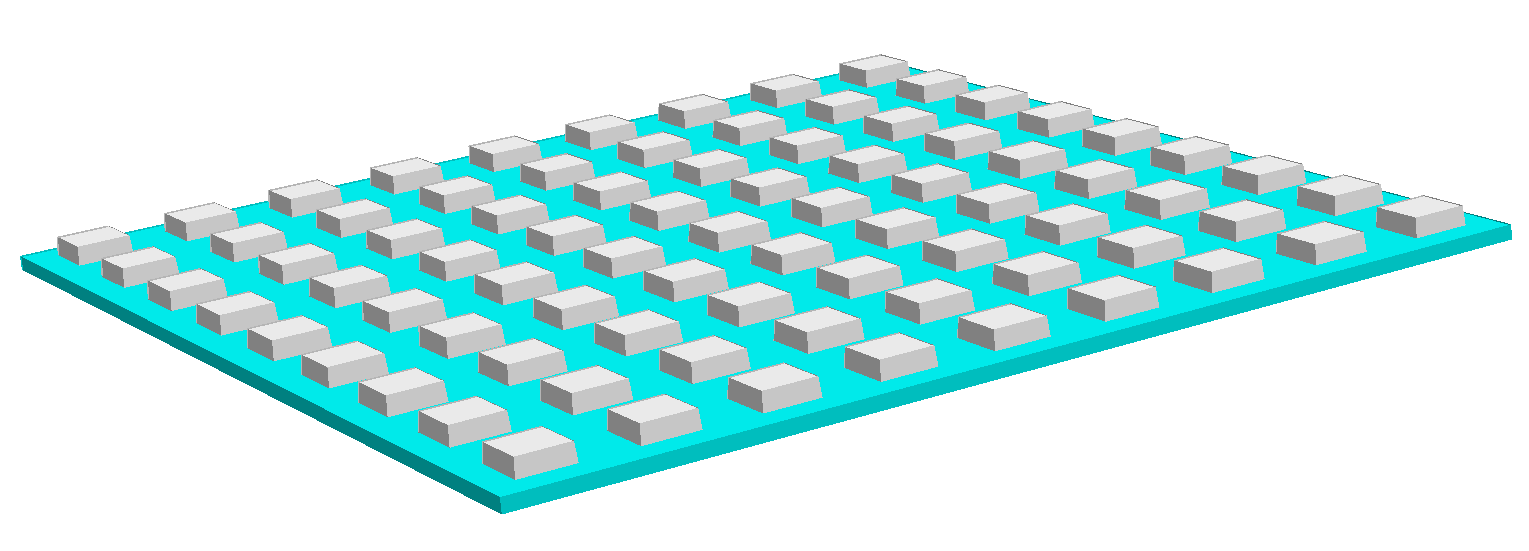# Gratings¶

An optical grating is a periodical structure for the diffraction of light. It can diffract an incoming light beam into several beam travelling in different directions.

1D grating

A 1D grating is periodic in one direction with lattice vectorand geometrically invariant in the-direction (this follows the JCMsuite convention that the coordinate system for 2D setups is theplane),Due to-invariance the structure appears as lines in 3D:

Numerically, the scattering problem reduces to a periodic unit cell of a 2D computational domain, see example Line Grating.

2D grating

A 2D grating is periodic in both horizontal directions. There exist two lattice vectorsso that the geometry is when shifted by a lattice vector,The following figures show a square lattice and a hexagonal lattice arrangement.Square lattice arrangement.are orthogonal.Hexagonal lattice arrangement. The lattice vectorsform an angle ofand are of equal length.

For the simulation it is possible to restrict the computation on a unit cell (primitive unit cell), see examples Square Unit Cell and Hexagonal Unit Cell.